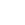• Articles coming soon
• Articles coming soon
• Articles coming soon
• Articles coming soon

# Changing the Room Settings

For correct PPFD and DLI calculations, some parameters of the room need to be entered.

PPFD is the PPF per square meter. It is often more logical to specify the amount of light in terms of photons that the plant receives rather than the number of photons the fixture emits, which is what PPFD does.

DLI is calculation-wise one step further and expresses the number of photons the plant receives per day cycle rather than per second.

### To use these power units:

• Enter the menu• Enter Preferences,,,
• Go to Power unit,• Change the value to PPF or PPFD,When the schedule is inactive, the DLI is equal to PPFD times 86400 (the number of seconds in a day). The PPFD is displayed in µmol/s/m2 whereas DLI is specified in mol/d/m2 (factor of 1,000,000 difference)### To configure the room:

• Enter the menu,• Go to Room settings,,• Change the values of Area W and Area L to match the surface area illuminated by this fixture (usually the gird spacing) ,,• Change the value of Correction to adjust for differences between expected and measured PPFD values

The correction factor accounts for wall (reflection) losses and out-of-bound losses (illuminating the path rather than the plants). The default is 80%.

### How to calibrate the Correction factor:

• Get a hold of a light meter capable of measuring PAR values, fitted with a cosine-corrected light collector
• Set the fixture’s power unit to PPFD
• Set the Correction value to 100%
• Turn on the outputs and take note of the displayed PPFD value. Call this PPFD1
• Using the PAR meter, measure the PPFD (400‑700nm) at soil level, unobscured by anything like the canopy. Measure in, or convert the unit to µmol/s/m2 (note1). Call this PPFD2
• Calculate PPFD2÷PPFD1×100%. This value should be less than 100% if done right
• Enter this value in the Correction setting

Note1) Different representations of the same unit include µmol/m2/s, µmol/s·m2, and µmol s−1 m−1.